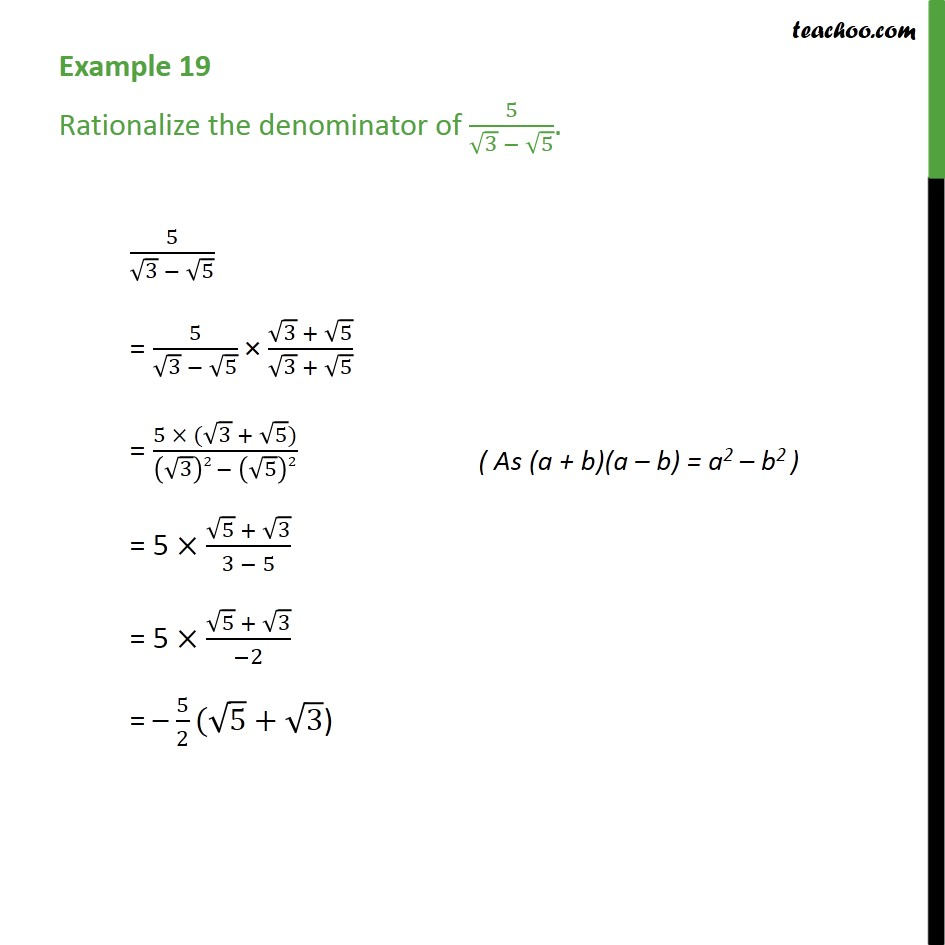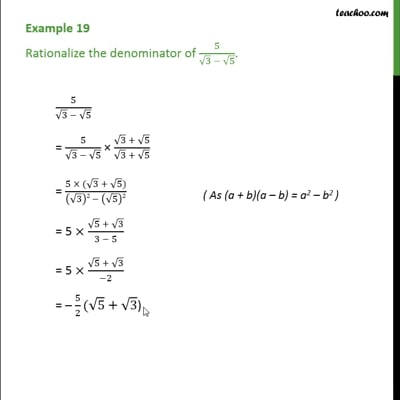Examples

Chapter 1 Class 9 Number Systems
Serial order wiseThis video is only available for Teachoo black users

Get live Maths 1-on-1 Classs - Class 6 to 12

### Transcript

Example 19 Rationalize the denominator of 5/(√3 − √5). 5/(√3 − √5) = 5/(√3 − √5) × (√3 + √5)/(√3 + √5) = (5 × (√3 + √5))/((√3)2 − (√5)2) = 5 ×(√5 + √3)/(3 − 5) = 5 ×(√5 + √3)/(−2) = – 5/2(√5+√3)